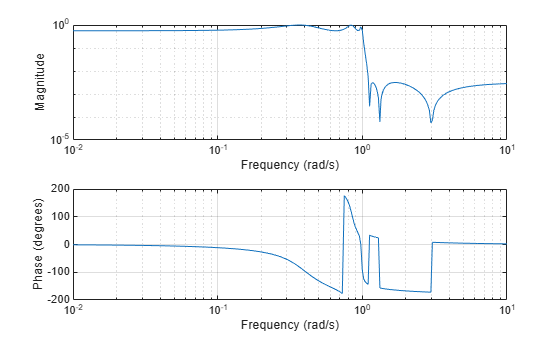# ellipap

Elliptic analog lowpass filter prototype

## Syntax

``[z,p,k] = ellipap(n,Rp,Rs)``

## Description

example

````[z,p,k] = ellipap(n,Rp,Rs)` returns the zeros, poles, and gain of an `n`th-order elliptic analog lowpass filter prototype, with `Rp` dB of ripple in the passband and a stopband `Rs` dB down from the peak value in the passband.```

## Examples

collapse all

Design a 6th-order elliptic analog lowpass filter with 5 dB of ripple in the passband and 50 dB of stopband attenuation.

`[z,p,k] = ellipap(6,5,50);`

Convert the zero-pole-gain filter parameters to transfer function form and display the frequency response of the filter.

```[b,a] = zp2tf(z,p,k); freqs(b,a)```## Input Arguments

collapse all

Filter order, specified as a positive integer scalar.

Data Types: `double`

Passband ripple, specified as a positive scalar in decibels.

Data Types: `double`

Stopband attenuation down from the peak passband value, specified as a positive scalar in decibels.

Data Types: `double`

## Output Arguments

collapse all

Zeros of the filter, returned as a column vector of length `n`. If `n` is odd, then `z` has a length equal to `n` – 1.

Poles of the filter, returned as a column vector of length `n`.

Gain of the filter, returned as a scalar.

## Algorithms

The `ellipap` function uses the algorithm outlined in . It employs `ellipke` to calculate the complete elliptic integral of the first kind and `ellipj` to calculate Jacobi elliptic functions. The function sets the passband edge angular frequency ω0 of the elliptic filter to 1 for a normalized result. The passband edge angular frequency is the frequency at which the passband ends and the filter has a magnitude response of 10-Rp/20.

The transfer function in factored zero-pole form is

`$H\left(s\right)=\frac{z\left(s\right)}{p\left(s\right)}=k\frac{\left(s-{z}_{1}\right)\left(s-{z}_{2}\right)\dots \left(s-{z}_{N}\right)}{\left(s-{p}_{1}\right)\left(s-{p}_{2}\right)\dots \left(s-{p}_{M}\right)}$`

Elliptic filters offer steeper rolloff characteristics than Butterworth and Chebyshev filters, but they are equiripple in both the passband and the stopband. Of the four classical filter types, elliptic filters usually meet a given set of filter performance specifications with the lowest filter order.

 Parks, T. W., and C. S. Burrus. Digital Filter Design. New York: John Wiley & Sons, 1987, chap. 7.